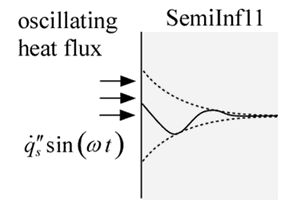Contents - Index

Sinusoidally Varying Heat Flux at the Surface of a Semi-Infinite BodyFunction SemiInf11(T_i, alpha, k, q''_dot_s, omega, x, time) returns the temperature within a semi-infinite body subjected to a sinusoidally varying surface heat flux.  The quasi-steady temperature distribution is returned.

The calling protocol is:

T = SemiInf11(T_i, alpha, k, q``_dot_s, omega, x, time)

Inputs:
T_i = temperature far from the surface [C] or [K]
alpha = thermal diffusivity [m^2/s]
k = thermal conductivity [W/m-K]
q``_dot_s = amplitude of surface heat flux [W/m^2]
omega = angular frequency of heat flux variation [rad/s] or [degree/s]
x = position relative to surface [m]
time = time relative to beginning of heat flux cycle [s]

This function can be used with English units set in EES.  In this case, T_i is in [F] or [R], alpha is in [ft^2/hr], k is in [Btu/hr-ft-R], q``_dot_s is in [Btu/hr-ft^2], x is in [ft], and time is in [s].

Outputs:
T is the temperature in [C] or [K] (or [F] or [R] in English units)

Carslaw, H.S. and J.C. Jaeger, Conduction of Heat in Solids, 2nd Edition, Oxford, (1959).

Example:
\$UnitSystem SI Mass J K Pa Radian

T_i=300 [K]
alpha=1e-3 [m^2/s]
k=1 [W/m-K]
q``_dot_s=1e4 [W/m^2]